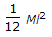# Mechanical Engineering - Engineering Mechanics

### Exercise :: Engineering Mechanics - Section 1

36.

The angle between two forces when the resultant is maximum and minimum respectively are

 A. 0° and 180° B. 180° and 0° C. 90° and 180° D. 90° and 0°

Explanation:

No answer description available for this question. Let us discuss.

37.

The path of the projectile is a parabola.

 A. True B. False

Explanation:

No answer description available for this question. Let us discuss.

38.

The equivalent length of a simple pendulum which gives the same frequency as compound pendulum is

 A.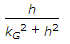B.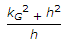C.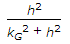D.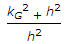Explanation:

No answer description available for this question. Let us discuss.

39.

A redundant frame is also called __________ frame.

 A. perfect B. imperfect C. deficient

Explanation:

No answer description available for this question. Let us discuss.

40.

Mass moment of inertia of a uni form thin rod of mass M and length (l) about its mid-point and perpendicular to its length is

 A.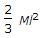B.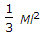C.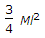D.